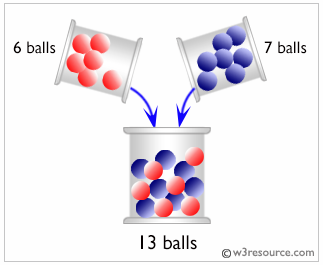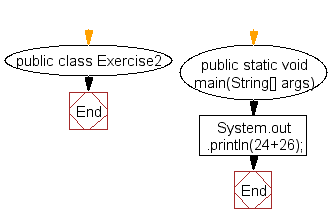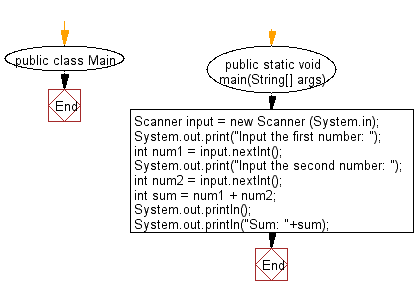﻿ Java exercises: Print the sum of two numbers - w3resource# Java Exercises: Print the sum of two numbers

## Java Basic: Exercise-2 with Solution

Write a Java program to print the sum of two numbers.

In mathematics, summation (capital Greek sigma symbol: ∑) is the addition of a sequence of numbers; the result is their sum or total. The numbers to be summed may be integers, rational numbers, real numbers, or complex numbers.

Pictorial Presentation:Sample Solution:

Java Code:

``````public class Exercise2 {

public static void main(String[] args) {
System.out.println(24+26);
}

}
```
```

Sample Output:

```50
```

Flowchart:Sample solution using input from the user:

Java Code:

``````import java.util.Scanner;

public class Main {
public static void main(String[] args)
{
Scanner input = new Scanner (System.in);
System.out.print("Input the first number: ");
int num1 = input.nextInt();
System.out.print("Input the second number: ");
int num2 = input.nextInt();
int sum = num1 + num2;
System.out.println();
System.out.println("Sum: "+sum);
}
}``````

Sample Output:

```Input the first number:  256
Input the second number:  326

Sum: 582
```

Flowchart:Java Code Editor:

What is the difficulty level of this exercise?

Test your Programming skills with w3resource's quiz.

﻿

## Java: Tips of the Day

countOccurrences

Counts the occurrences of a value in an array.

Use Arrays.stream().filter().count() to count total number of values that equals the specified value.

```public static long countOccurrences(int[] numbers, int value) {
return Arrays.stream(numbers)
.filter(number -> number == value)
.count();
}
```

Ref: https://bit.ly/3kCAgLb